Updating search results...

# 18 Results

View
Selected filters:
• Distributive Property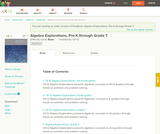Unrestricted Use
CC BY
Rating
0.0 stars

CK-12 Algebra Explorations is a hands-on series of activities that guides students from Pre-K to Grade 7 through algebraic concepts.

Subject:
Algebra
Functions
Material Type:
Activity/Lab
Lesson Plan
Textbook
Provider:
CK-12 Foundation
Provider Set:
CK-12 FlexBook
Author:
Mary Cavanagh, Carol Findell, Carole Greenes
Date Added:
10/04/2011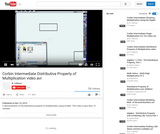Read the Fine Print
Rating
4.0 stars

A demonstration of the distributive property of multiplication using models. This video is less than 15 minutes.

Subject:
Numbers and Operations
Material Type:
Lecture
Provider:
Individual Authors
Provider Set:
Individual Authors
Author:
nlc464
Date Added:
04/11/2013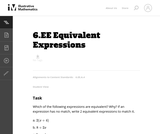Unrestricted Use
CC BY
Rating
0.0 stars

In this problem students must transform expressions using the distributive, commutative and associative properties to decide which expressions are equivalent.

Subject:
Mathematics
Material Type:
Activity/Lab
Provider:
Illustrative Mathematics
Provider Set:
Illustrative Mathematics
Author:
Illustrative Mathematics
Date Added:
05/01/2012Unrestricted Use
CC BY
Rating
0.0 stars

Math unit about understanding Perimeter and Area using Khan Academy materials. Appropriate for grades 3-5.

Subject:
Mathematics
Material Type:
Assessment
Homework/Assignment
Lesson Plan
Author:
Amy Whitchurch
Date Added:
01/28/2016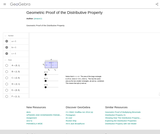Conditional Remix & Share Permitted
CC BY-NC-SA
Rating
0.0 stars

This resource demonstrates the distributive property through the area of rectangles. You can manipulate the dimension by moving the slider.

Subject:
Mathematics
Algebra
Geometry
Material Type:
Diagram/Illustration
Simulation
Provider:
GeoGebra
Author:
jtmace11
Date Added:
05/10/2018Only Sharing Permitted
CC BY-NC-ND
Rating
4.0 stars

This lesson unit is intended to help you assess how well students are able to: Perform arithmetic operations, including those involving whole-number exponents, recognizing and applying the conventional order of operations; Write and evaluate numerical expressions from diagrammatic representations and be able to identify equivalent expressions; apply the distributive and commutative properties appropriately; and use the method for finding areas of compound rectangles.

Subject:
Geometry
Material Type:
Assessment
Lesson Plan
Provider:
Shell Center for Mathematical Education
Provider Set:
Mathematics Assessment Project (MAP)
Date Added:
04/26/2013Conditional Remix & Share Permitted
CC BY-NC
Rating
0.0 stars

Subject:
Mathematics
Material Type:
Full Course
Provider:
Pearson
Date Added:
10/06/2016Rating
0.0 stars

Expressions

Type of Unit: Concept

Prior Knowledge

Students should be able to:

Write and evaluate simple expressions that record calculations with numbers.
Use parentheses, brackets, or braces in numerical expressions and evaluate expressions with these symbols.
Interpret numerical expressions without evaluating them.

Lesson Flow

Students learn to write and evaluate numerical expressions involving the four basic arithmetic operations and whole-number exponents. In specific contexts, they create and interpret numerical expressions and evaluate them. Then students move on to algebraic expressions, in which letters stand for numbers. In specific contexts, students simplify algebraic expressions and evaluate them for given values of the variables. Students learn about and use the vocabulary of algebraic expressions. Then they identify equivalent expressions and apply properties of operations, such as the distributive property, to generate equivalent expressions. Finally, students use geometric models to explore greatest common factors and least common multiples.

Subject:
Mathematics
Algebra
Material Type:
Unit of Study
Provider:
PearsonConditional Remix & Share Permitted
CC BY-NC
Rating
0.0 stars

Students use a rectangular area model to understand the distributive property. They watch a video to find how to express the area of a rectangle in two different ways. Then they find the area of rectangular garden plots in two ways.Key ConceptsThe distributive property can be used to rewrite an expression as an equivalent expression that is easier to work with. The distributive property states that multiplication distributes over addition.Applying multiplication to quantities that have been combined by addition: a(b + c)Applying multiplication to each quantity individually, and then adding the products together: ab + acThe distributive property can be represented with a geometric model. The area of this rectangle can be found in two ways: a(b + c) or ab + ac. The equality of these two expressions, a(b + c) = ab + ac, is the distributive property.Goals and Learning ObjectivesUse a geometric model to understand the distributive property.Write equivalent expressions using the distributive property.

Subject:
Algebra
Geometry
Material Type:
Lesson Plan
Provider:
Pearson
Date Added:
09/21/2015Conditional Remix & Share Permitted
CC BY-NC
Rating
0.0 stars

Four full-year digital course, built from the ground up and fully-aligned to the Common Core State Standards, for 7th grade Mathematics. Created using research-based approaches to teaching and learning, the Open Access Common Core Course for Mathematics is designed with student-centered learning in mind, including activities for students to develop valuable 21st century skills and academic mindset.

Subject:
Mathematics
Material Type:
Full Course
Provider:
Pearson
Date Added:
10/06/2016Rating
0.0 stars

Algebraic Reasoning

Type of Unit: Concept

Prior Knowledge

Students should be able to:

Add, subtract, multiply, and divide rational numbers.
Evaluate expressions for a value of a variable.
Use the distributive property to generate equivalent expressions including combining like terms.
Understand solving an equation or inequality as a process of answering a question: which values from a specified set, if any, make the equation or inequality true?
Write and solve equations of the form x+p=q and px=q for cases in which p, q, and x are non-negative rational numbers.
Understand and graph solutions to inequalities x<c or x>c.
Use equations, tables, and graphs to represent the relationship between two variables.
Relate fractions, decimals, and percents.
Solve percent problems included those involving percent of increase or percent of decrease.

Lesson Flow

This unit covers all of the Common Core State Standards for Expressions and Equations in Grade 7. Students extend what they learned in Grade 6 about evaluating expressions and using properties to write equivalent expressions. They write, evaluate, and simplify expressions that now contain both positive and negative rational numbers. They write algebraic expressions for problem situations and discuss how different equivalent expressions can be used to represent different ways of solving the same problem. They make connections between various forms of rational numbers. Students apply what they learned in Grade 6 about solving equations such as x+2=6 or 3x=12 to solving equations such as 3x+6=12 and 3(x−2)=12. Students solve these equations using formal algebraic methods. The numbers in these equations can now be rational numbers. They use estimation and mental math to estimate solutions. They learn how solving linear inequalities differs from solving linear equations and then they solve and graph linear inequalities such as −3x+4<12. Students use inequalities to solve real-world problems, solving the problem first by arithmetic and then by writing and solving an inequality. They see that the solution of the algebraic inequality may differ from the solution to the problem.

Subject:
Mathematics
Algebra
Material Type:
Unit of Study
Provider:
PearsonConditional Remix & Share Permitted
CC BY-NC
Rating
0.0 stars

Students use the distributive property to simplify expressions. Simplifying expressions may include multiplying by a negative number. Students analyze and identify errors that are sometimes made when simplifying expressions.Key ConceptsThis lesson focuses on simplifying expressions and requires an understanding of the rules for multiplying negative numbers. For example, students simplify expressions such as 8 − 3(2 − 4x). These kinds of expressions are often difficult for students because there are several errors that they can make based on misconceptions:Students may simplify 8 − 3(2 − 4x) to 5(2 − 4x) because they mistakenly detach the 3 from the multiplication.Students may simplify 8 − 3(2 − 4x) to 8 − 3(−2x) in an attempt to simplify the expression in parentheses even though no simplification is possible.Students may simplify 8 − 3(2 − 4x) to 8 − 6 −12x. This error could be based on a misunderstanding of how the distributive property works or on lack of knowledge of the rules for multiplying integers.Goals and Learning ObjectivesSimplify more complicated expressions that involve multiplication by negative numbers.Identify errors that can be made when simplifying expressions.

Subject:
Algebra
Material Type:
Lesson Plan
Provider:
Pearson
Date Added:
09/21/2015Rating
0.0 stars

Proportional Relationships

Type of Unit: Concept

Prior Knowledge

Students should be able to:

Understand what a rate and ratio are.
Make a ratio table.
Make a graph using values from a ratio table.

Lesson Flow

Students start the unit by predicting what will happen in certain situations. They intuitively discover they can predict the situations that are proportional and might have a hard time predicting the ones that are not. In Lessons 2–4, students use the same three situations to explore proportional relationships. Two of the relationships are proportional and one is not. They look at these situations in tables, equations, and graphs. After Lesson 4, students realize a proportional relationship is represented on a graph as a straight line that passes through the origin. In Lesson 5, they look at straight lines that do not represent a proportional relationship. Lesson 6 focuses on the idea of how a proportion that they solved in sixth grade relates to a proportional relationship. They follow that by looking at rates expressed as fractions, finding the unit rate (the constant of proportionality), and then using the constant of proportionality to solve a problem. In Lesson 8, students fine-tune their definition of proportional relationship by looking at situations and determining if they represent proportional relationships and justifying their reasoning. They then apply what they have learned to a situation about flags and stars and extend that thinking to comparing two prices—examining the equations and the graphs. The Putting It Together lesson has them solve two problems and then critique other student work.

Gallery 1 provides students with additional proportional relationship problems.

The second part of the unit works with percents. First, percents are tied to proportional relationships, and then students examine percent situations as formulas, graphs, and tables. They then move to a new context—salary increase—and see the similarities with sales taxes. Next, students explore percent decrease, and then they analyze inaccurate statements involving percents, explaining why the statements are incorrect. Students end this sequence of lessons with a formative assessment that focuses on percent increase and percent decrease and ties it to decimals.

Students have ample opportunities to check, deepen, and apply their understanding of proportional relationships, including percents, with the selection of problems in Gallery 2.

Subject:
Mathematics
Ratios and Proportions
Material Type:
Unit of Study
Provider:
PearsonConditional Remix & Share Permitted
CC BY-NC
Rating
0.0 stars

Students represent and solve percent decrease problems.Key ConceptsWhen there is a percent decrease between a starting amount and a final amount, the relationship can be represented by an equation of the form y = kx where y is the final amount, x is the starting amount, and k is the constant of proportionality, which is equal to 1 minus the percent change, p, represented as a decimal: k = 1 – p, so y = (1 – p)x.The constant of proportionality k has the value it does—a number less than 1—because of the way the distributive property can be used to simplify the expression for the starting amount decreased by a percent of the starting amount: x – x(p) = x(1 – p).Goals and Learning ObjectivesDetermine the unknown amount—either the starting amount, the percent change, or the final amount—in a percent decrease situation when given the other two amounts.Make a table to represent a percent decrease problem.Write and solve an equation to represent a percent decrease problem.

Subject:
Ratios and Proportions
Material Type:
Lesson Plan
Provider:
Pearson
Date Added:
09/21/2015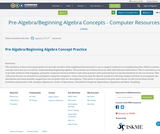Conditional Remix & Share Permitted
CC BY-NC-SA
Rating
4.0 stars

The intention of this curriculum guide is to provide teachers with supplemental materials to use to support students in strengthening their skills in various concept areas that are crucial for understanding beginning algebra. The activities are broken down by skill with links provided below. This is intended as a way to provide students with engaging, primarily computer-based activities to get extra practice with material that is covered elsewhere in the curriculum. This collection focuses on simulations and games using the computer—some resources may be ripe for teachers to develop unique activities to accompany the simulation and some possible suggestions are included with the descriptions. This series is intended to be pick-and-choose.

In this Curriculum Guide:

Activities and practice with: Integers, Exponents, Order of Operations, Distributive Property, Expressions, Equations and Basic Graphing

Subject:
Mathematics
Material Type:
Activity/Lab
Diagram/Illustration
Game
Interactive
Simulation
Author:
Lee Gargagliano
Date Added:
05/24/2018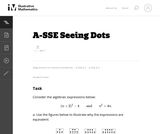Unrestricted Use
CC BY
Rating
4.0 stars

The purpose of this task is to identify the structure in the two algebraic expressions by interpreting them in terms of a geometric context. Students will have likely seen this type of process before, so the principal source of challenge in this task is to encourage a multitude and variety of approaches, both in terms of the geometric argument and in terms of the algebraic manipulation.

Subject:
Mathematics
Algebra
Material Type:
Activity/Lab
Provider:
Illustrative Mathematics
Provider Set:
Illustrative Mathematics
Author:
Illustrative Mathematics
Date Added:
05/17/2013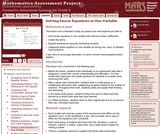Only Sharing Permitted
CC BY-NC-ND
Rating
5.0 stars

This lesson unit is intended to help teachers assess how well students are able to: solve linear equations in one variable with rational number coefficients; collect like terms; expand expressions using the distributive property; and categorize linear equations in one variable as having one, none, or infinitely many solutions. It also aims to encourage discussion on some common misconceptions about algebra.

Subject:
Algebra
Material Type:
Assessment
Lesson Plan
Provider:
Shell Center for Mathematical Education
Provider Set:
Mathematics Assessment Project (MAP)
Date Added:
04/26/2013